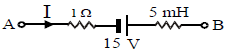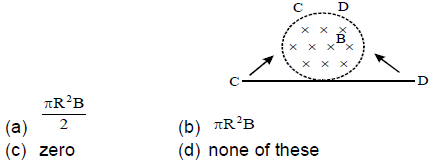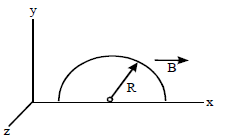# JEE Main Sample Paper 2015 Set B

JEE Main Sample Paper 2015 Set B. Please see the link below for the sample paper for the upcoming JEE (Main) examination which is conducted by CBSE for candidates to get admission into various engineering colleges like NITs and IIITs and other affiliated colleges all over India. Candidates are suggested to download the sample paper/ model test paper and practice the questions for the upcoming JEE (Main) examinations.

PHYSICS

1. A metal rod moves at a constant velocity in a direction perpendicular to its length. A constant, uniform magnetic field exists in space in a direction perpendicular to the rod as well as its velocity. Select the correct statement from the following

(a) the entire rod is at the same electric potential
(b) there is an electric field in the rod
(c) the electric potential is highest at the centre for the rod and decreases towards at its ends
(d) the electric potential is lowest at the centre of the rod, and increases towards its ends.

2. A small circular loop of radius r is placed inside a circular loop of radius R (R >> r).The loops are coplanar and their centers coincide. The mutual inductance of the system is proportional to

(a) r/R
(b) r2/R
(c) r/R2
(d) r2/R2

3. The figure shows a part of a complete circuit. The potential difference VB – VA when the current I is 5A and is decreasing at a rate of 103 As–1 is given by4. A train travelling with the speed of 90 km/hr at the north pole has axle of length 1m.If earth’s magnetic field at the north pole has magnitude of 5 × 10–4 T. The induced emf in the rod is

(a) 6.25 mV
(b) 12.50 mV
(c) 25.50 mV
(d) zero

5. A bar magnet is moved between two parallel circular loops A and B with a constant velocity v as shown in figure. Then(a) The current in each loop flows in the same direction
(b) The current in each loop flows in the opposite direction
(c) The loops will repel each other
(d) The loops will vibrate.

6. A time – varying magnetic field produces an induced electric field E, then which of the following statement is wrong

(a) E is a non-conservative field
(b) The potential of E is not defined
(c) Field lines of E form closed loops
(d) The field E is electrostatic

7. The figure shows a straight wire lying in the plane of the paper and a uniform magnetic field perpendicular to the plane of the paper. The ends C and D are slowly turned to form a ring of radius R so that the entire magnetic field is confined in it. The emf induced in the ring is given by8. A semicircle conducting ring of radius R is radius in the xy plane, as shown in the figure. A uniform magnetic field is set up along the x-axis. No emf, will be induced in the ring, if(a) it moves along the x-axis
(b) it moves along the y-axis
(c) it moves along the z-axis
(d) all of these

9. There is increasing magnetic field through a conducting ring as shown in figure. The direction of induced current as seen from above the plane of coil is(a) clockwise
(b) anticlockwise
(c) no induced current
(d) first clockwise then anticlockwise

Refer to link below JEE Main Sample Paper 2015 Set B